# 2.内容

• 基于内存：主要通过计算近似度来进行推荐，比如基于用户（Used-Based）和基于物品（Item-Based）的协同过滤，这两个模式中都会首先构建用户交互矩阵，然后矩阵的行向量和列向量可以用来表示用户和物品，然后计算用户和物品的相似度来进行推荐；
• 基于模型：主要是对交互矩阵进行填充，预测用户购买某个物品的可能性。

• pandas
• numpy
• sklearn

Python环境：

• 版本3.7.6
• Anaconda3

## 2.2 评分函数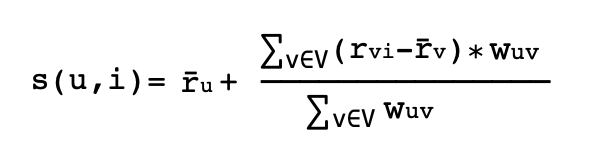这里其中s为预测得分，u为用户，i为物品，r为用户给出的评分，w为权重。在这种情况下，我们的分数等于每个用户对该项目的评价减去该用户的平均评价再乘以某个权重的总和，这个权重表示该用户与其他用户有多少相似之处，或者对其他用户的预测有多少贡献。这是用户u和v之间的权重，分数在0到1之间，其中0是最低的，1是最高的。理论上看起来非常完美，那为啥需要从每个用户的评分中减去平均评分，为啥要使用加权平均而不是简单平均？这是因为我们所处理的用户类型，首先，人们通常在不同的尺度上打分，用户A可能是一个积极乐观的用户，会给用户A自己喜欢的电影平均高分（例如4分、或者5分）。而用户B是一个不乐观或者对评分标准比较高的用户，他可能对最喜欢的电影评分为2分到5分之间。用户B的2分对应到用户A的4分。改进之处是可以通过规范化用户评分来提高算法效率。一种方法是计算s(u,i)的分数，它是用户对每件物品的平均评价加上一些偏差。通过使用余弦相似度来计算上述公式中给出的权重，同时，按照上述方式对数据进行归一化，在pandas中进行一些数据分析。

### 2.2.1 导入Python依赖包

import pandas as pd
import numpy as np
from sklearn.metrics.pairwise import cosine_similarity
from sklearn.metrics import pairwise_distances

### 2.2.2 加载数据源

movies = pd.read_csv("data/movies.csv")
Tags = pd.read_csv("data/tags.csv")

print(movies.head())
print(Tags.head())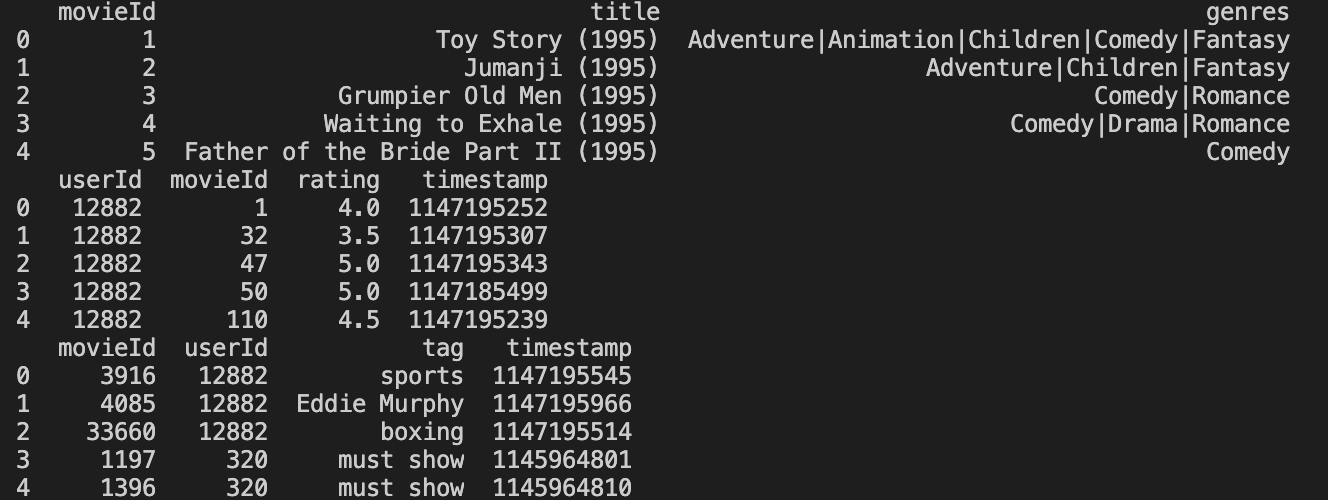构建数据：

Mean = Ratings.groupby(by="userId", as_index=False)['rating'].mean()
Rating_avg = pd.merge(Ratings, Mean, on='userId')
print(Rating_avg.head())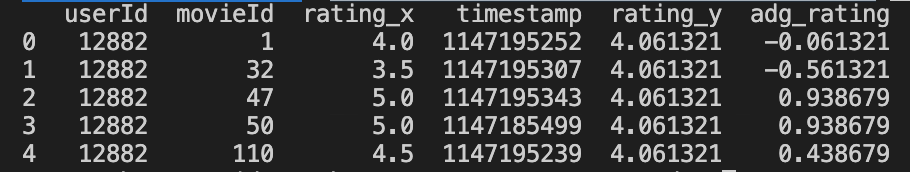## 2.3 余弦相似度

对于上面的公式，我们需要找到有相似想法的用户。找到一个喜欢和不喜欢的用户听起来很有意思，但是我们如何找到相似性呢？那么这里我们就需要用到余弦相似度，看看用户有多相似。它通常是根据用户过去的评分来计算的。

check = pd.pivot_table(Rating_avg,values='rating_x',index='userId',columns='movieId')
print(final.head())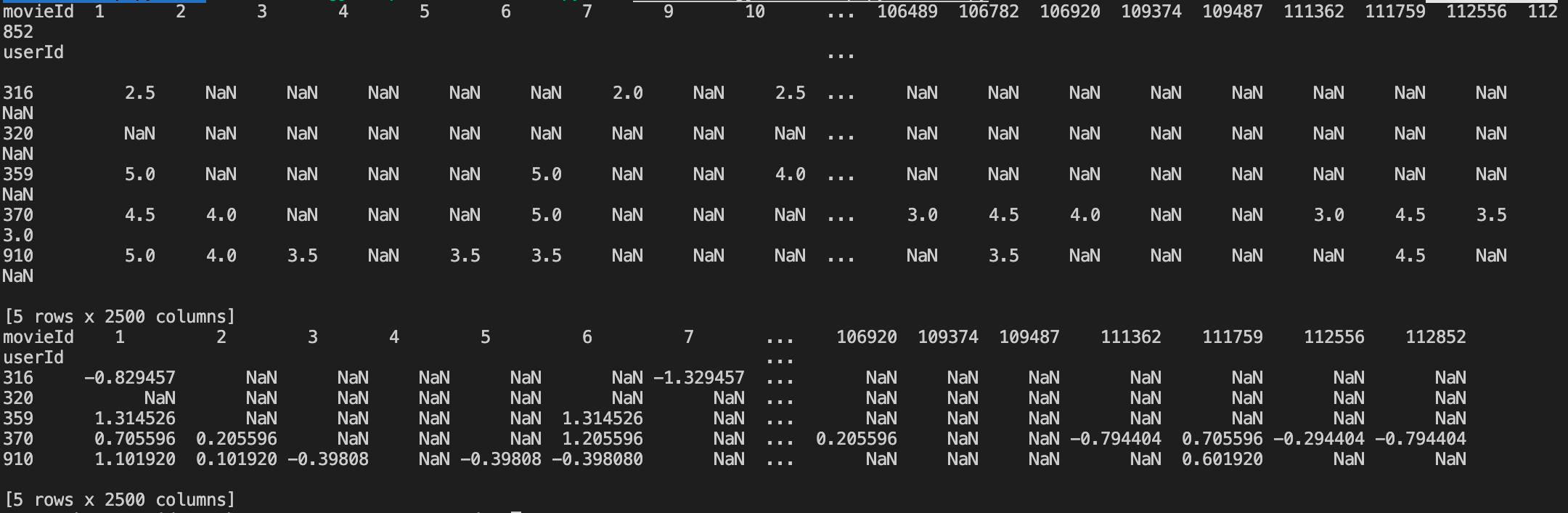1. 使用行上的用户平均值；
2. 用户在列上的电影平均值

# Replacing NaN by Movie Average
final_movie = final.fillna(final.mean(axis=0))

# Replacing NaN by user Average
final_user = final.apply(lambda row: row.fillna(row.mean()), axis=1)
print(final_user.head())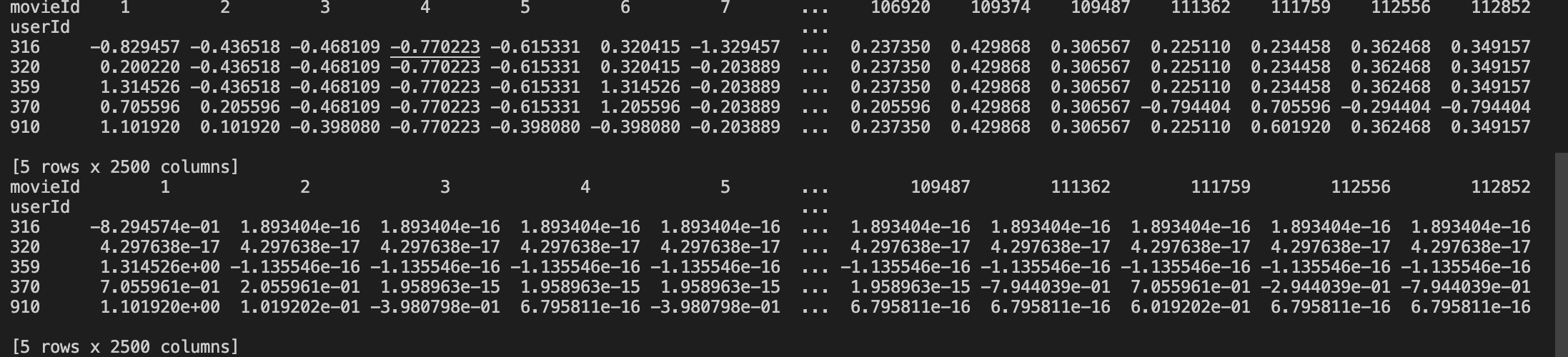接着，我们开始计算用户之间的相似性，代码如下：

# user similarity on replacing NAN by item(movie) avg
cosine = cosine_similarity(final_movie)
np.fill_diagonal(cosine, 0)
similarity_with_movie = pd.DataFrame(cosine, index=final_movie.index)
similarity_with_movie.columns = final_user.index

# user similarity on replacing NAN by user avg
b = cosine_similarity(final_user)
np.fill_diagonal(b, 0 )
similarity_with_user = pd.DataFrame(b,index=final_user.index)
similarity_with_user.columns=final_user.index
# print(similarity_with_user.head())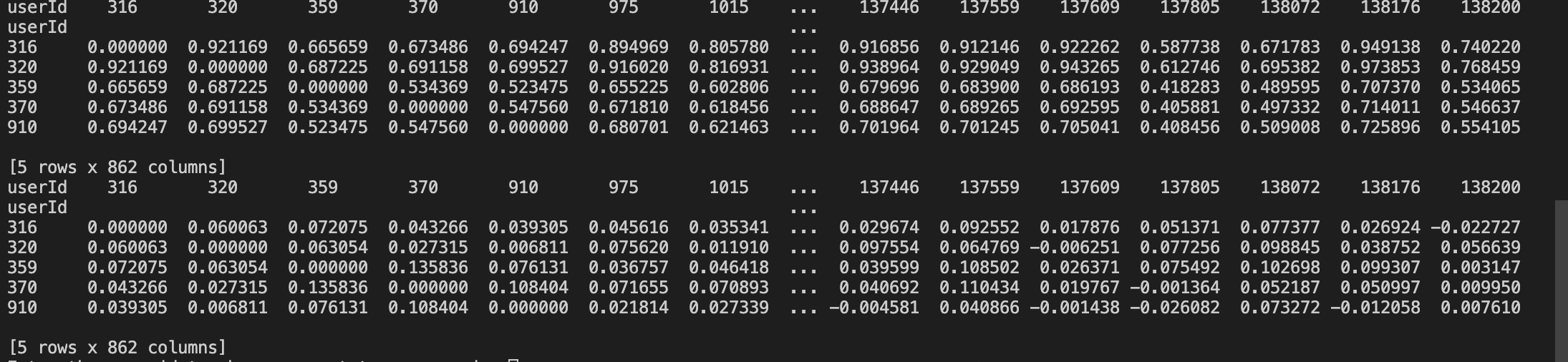然后，我们来检验一下我们的相似度是否有效，代码如下：

def get_user_similar_movies( user1, user2 ):
common_movies = Rating_avg[Rating_avg.userId == user1].merge(
Rating_avg[Rating_avg.userId == user2],
on = "movieId",
how = "inner" )
return common_movies.merge( movies, on = 'movieId' )

a = get_user_similar_movies(370,86309)
a = a.loc[ : , ['rating_x_x','rating_x_y','title']]
print(a.head())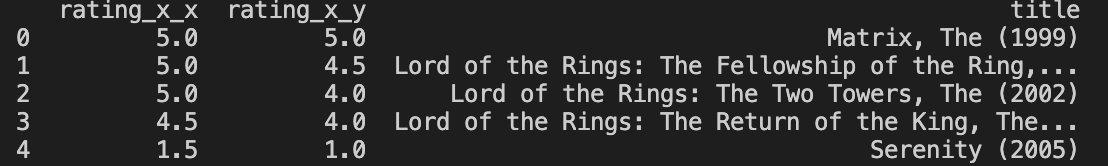从上图中，我们可以看出产生的相似度几乎是相同的，符合真实性。

## 2.4 相邻用户

def find_n_neighbours(df,n):
order = np.argsort(df.values, axis=1)[:, :n]
df = df.apply(lambda x: pd.Series(x.sort_values(ascending=False)
.iloc[:n].index,
index=['top{}'.format(i) for i in range(1, n+1)]), axis=1)
return df

# top 30 neighbours for each user
sim_user_30_u = find_n_neighbours(similarity_with_user,30)

sim_user_30_m = find_n_neighbours(similarity_with_movie,30)
print(sim_user_30_m.head())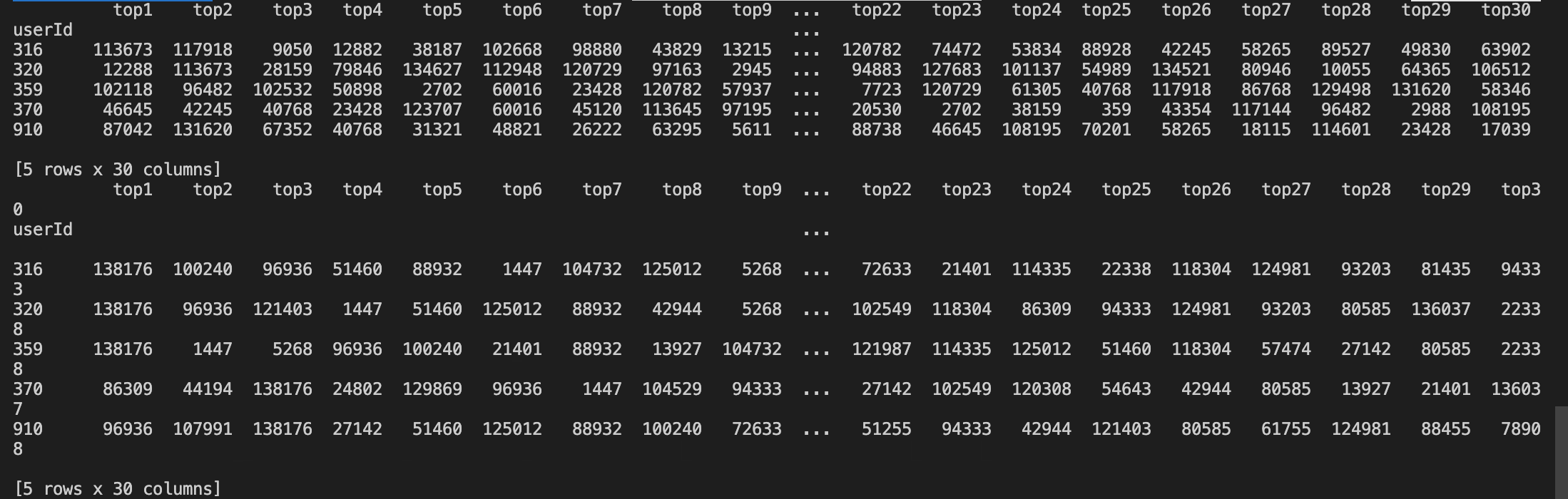## 2.5 计算最后得分

def User_item_score(user,item):
a = sim_user_30_m[sim_user_30_m.index==user].values
b = a.squeeze().tolist()
c = final_movie.loc[:,item]
d = c[c.index.isin(b)]
f = d[d.notnull()]
avg_user = Mean.loc[Mean['userId'] == user,'rating'].values
index = f.index.values.squeeze().tolist()
corr = similarity_with_movie.loc[user,index]
fin = pd.concat([f, corr], axis=1)
nume = fin['score'].sum()
deno = fin['correlation'].sum()
final_score = avg_user + (nume/deno)
return final_score

score = User_item_score(320,7371)
print("score (u,i) is",score)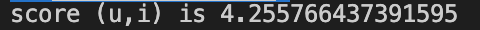Rating_avg = Rating_avg.astype({"movieId": str})
Movie_user = Rating_avg.groupby(by = 'userId')['movieId'].apply(lambda x:','.join(x))

def User_item_score1(user):
Movie_seen_by_user = check.columns[check[check.index==user].notna().any()].tolist()
a = sim_user_30_m[sim_user_30_m.index==user].values
b = a.squeeze().tolist()
d = Movie_user[Movie_user.index.isin(b)]
l = ','.join(d.values)
Movie_seen_by_similar_users = l.split(',')
Movies_under_consideration = list(set(Movie_seen_by_similar_users)-set(list(map(str, Movie_seen_by_user))))
Movies_under_consideration = list(map(int, Movies_under_consideration))
score = []
for item in Movies_under_consideration:
c = final_movie.loc[:,item]
d = c[c.index.isin(b)]
f = d[d.notnull()]
avg_user = Mean.loc[Mean['userId'] == user,'rating'].values
index = f.index.values.squeeze().tolist()
corr = similarity_with_movie.loc[user,index]
fin = pd.concat([f, corr], axis=1)
nume = fin['score'].sum()
deno = fin['correlation'].sum()
final_score = avg_user + (nume/deno)
score.append(final_score)
data = pd.DataFrame({'movieId':Movies_under_consideration,'score':score})
Movie_Name = top_5_recommendation.merge(movies, how='inner', on='movieId')
Movie_Names = Movie_Name.title.values.tolist()
return Movie_Names

user = int(input("Enter the user id to whom you want to recommend : "))
predicted_movies = User_item_score1(user)
print(" ")
print("The Recommendations for User Id : 370")
print("   ")
for i in predicted_movies:
print(i)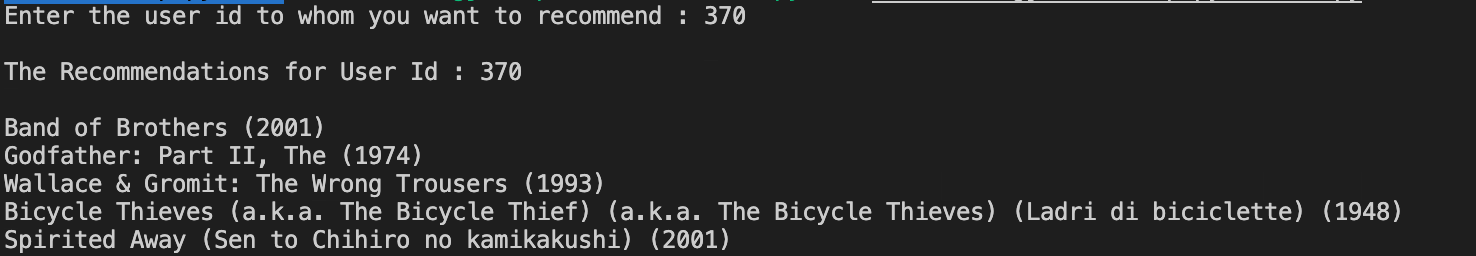# 3.总结

1. 采集数据 & 存储数据
2. 加载数据
3. 数据建模（数据预处理 & 数据清洗）
4. 计算相似性（余弦相似度、相邻计算）
5. 得分预测（预测和最终得分计算）
6. 物品推荐

# 4.结束语

QQ群（Kafka并不难学）： 825943084

### 转载请注明出处，谢谢合作！

posted @ 2020-06-25 13:54  哥不是小萝莉  阅读(1283)  评论(1编辑  收藏  举报